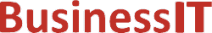O nás     Inzerce     KontaktSpolehlivé informace o IT již od roku 2011[Linux manuál]

# fdim, fdimf, fdiml: vypočítat kladný rozdíl mezi dvěma čísly s plovoucí desetinnou čárkou

Originální popis anglicky: fdim, fdimf, fdiml - compute positive difference between two floating-point numbers

Návod, kniha: POSIX Programmer's Manual

## STRUČNĚ

#include <math.h>

double fdim(double x, double y);

float fdimf(float x, float y);

long double fdiml(long double x, long double y);

## POPIS / INSTRUKCE

These functions shall determine the positive difference between their arguments. If x is greater than y, x- y is returned. If x is less than or equal to y, +0 is returned.
An application wishing to check for error situations should set errno to zero and call feclearexcept(FE_ALL_EXCEPT) before calling these functions. On return, if errno is non-zero or fetestexcept(FE_INVALID | FE_DIVBYZERO | FE_OVERFLOW | FE_UNDERFLOW) is non-zero, an error has occurred.

## NÁVRATOVÁ HODNOTA

Upon successful completion, these functions shall return the positive difference value.
If x- y is positive and overflows, a range error shall occur and fdim(), fdimf(), and fdiml() shall return the value of the macro HUGE_VAL, HUGE_VALF, and HUGE_VALL, respectively.
If x- y is positive and underflows, a range error may occur, and either ( x- y) (if representable),  or 0.0 (if supported), or an implementation-defined value shall be returned.
If x or y is NaN, a NaN shall be returned.

## CHYBY / ERRORY

The fdim() function shall fail if:
Range Error
The result overflows.
If the integer expression (math_errhandling & MATH_ERRNO) is non-zero, then errno shall be set to [ERANGE]. If the integer expression (math_errhandling & MATH_ERREXCEPT) is non-zero, then the overflow floating-point exception shall be raised.

The fdim() function may fail if:
Range Error
The result underflows.
If the integer expression (math_errhandling & MATH_ERRNO) is non-zero, then errno shall be set to [ERANGE]. If the integer expression (math_errhandling & MATH_ERREXCEPT) is non-zero, then the underflow floating-point exception shall be raised.

The following sections are informative.

None.

## APPLICATION USAGE

On implementations supporting IEEE Std 754-1985, x- y cannot underflow, and hence the 0.0 return value is shaded as an extension for implementations supporting the XSI extension rather than an MX extension.
On error, the expressions (math_errhandling & MATH_ERRNO) and (math_errhandling & MATH_ERREXCEPT) are independent of each other, but at least one of them must be non-zero.

None.

None.

## SOUVISEJÍCÍ

feclearexcept() , fetestexcept() , fmax() , fmin() , the Base Definitions volume of IEEE Std 1003.1-2001, Section 4.18, Treatment of Error Conditions for Mathematical Functions, <math.h> Portions of this text are reprinted and reproduced in electronic form from IEEE Std 1003.1, 2003 Edition, Standard for Information Technology -- Portable Operating System Interface (POSIX), The Open Group Base Specifications Issue 6, Copyright (C) 2001-2003 by the Institute of Electrical and Electronics Engineers, Inc and The Open Group. In the event of any discrepancy between this version and the original IEEE and The Open Group Standard, the original IEEE and The Open Group Standard is the referee document. The original Standard can be obtained online at http://www.opengroup.org/unix/online.html .
 2003 IEEE/The Open Group
©2011-2020 BusinessIT.cz, ISSN 1805-0522 | Názvy použité v textech mohou být ochrannými známkami příslušných vlastníků.
Provozovatel: Bispiral, s.r.o., kontakt: BusinessIT(at)Bispiral.com | Inzerce: Best Online Media, s.r.o., zuzana@online-media.cz
O vydavateli | Pravidla webu BusinessIT.cz a ochrana soukromí | pg(8859)